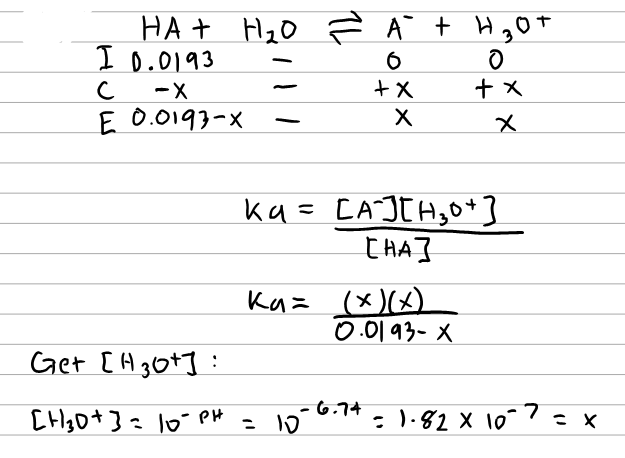# Problem: If enough of a monoprotic acid is dissolved in water to produce a 0.0193 M solution with a pH of 6.74, what is the equilibrium constant, pKa, for the acid?

###### FREE Expert Solution
81% (469 ratings)
###### FREE Expert Solution81% (469 ratings)###### Problem Details

If enough of a monoprotic acid is dissolved in water to produce a 0.0193 M solution with a pH of 6.74, what is the equilibrium constant, pKa, for the acid?

What scientific concept do you need to know in order to solve this problem?

Our tutors have indicated that to solve this problem you will need to apply the Weak Acids concept. If you need more Weak Acids practice, you can also practice Weak Acids practice problems.

What is the difficulty of this problem?

Our tutors rated the difficulty ofIf enough of a monoprotic acid is dissolved in water to prod...as medium difficulty.

How long does this problem take to solve?

Our expert Chemistry tutor, Sabrina took 8 minutes and 36 seconds to solve this problem. You can follow their steps in the video explanation above.

What professor is this problem relevant for?

Based on our data, we think this problem is relevant for Professor Roy's class at DUKE.